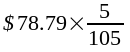SEARCH HOMEMath Central Quandaries & QueriesQuestion from Louise, a parent: I am doing our business books and could you please tell me how to figure out how to calculate what the 5% GST would be on a total. Such as in example.....$78.79 total figure.....what would be the GST portion. How do you figure it out. ?? ThanksHi Louise. If you think of the original price as 100% of the original price (sounds rather obvious, I know), and you add 5% tax, then your total is 105% of the original, right? Your total is$78.79, so that's the 105%. You are
interested in the tax portion (the 5%), so you just want the same proportion 5% is of 105%. Therefore, you just multiply it by that fraction:Punch that into your calculator to find the GST portion of the total.
Cheers,
Stephen La RocqueMath Central is supported by the University of Regina and The Pacific Institute for the Mathematical Sciences.